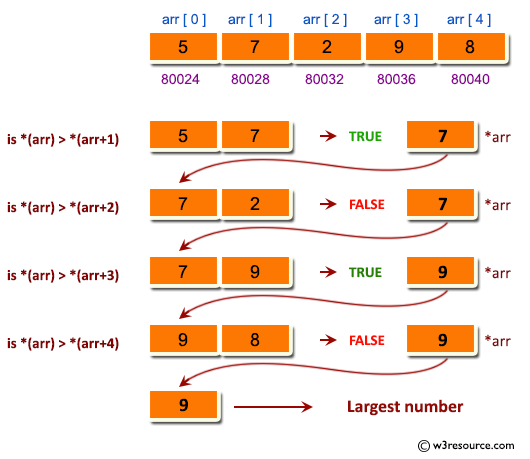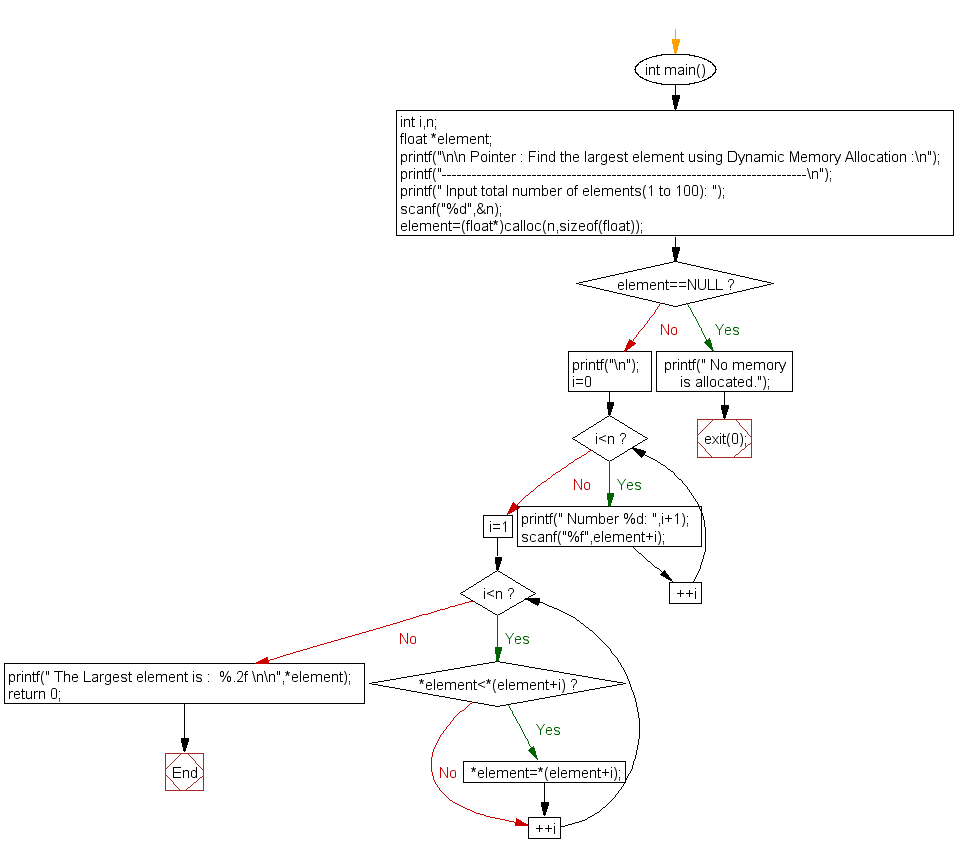﻿ C Program: Largest element by Dynamic Memory Allocation# C Exercises: Find the largest element using Dynamic Memory Allocation

## C Pointer : Exercise-9 with Solution

Write a program in C to find the largest element using Dynamic Memory Allocation.

Pictorial Presentation:Sample Solution:

C Code:

``````#include <stdio.h>
#include <stdlib.h>
int main()
{
int i,n;
float *element;
printf("\n\n Pointer : Find the largest element using Dynamic Memory Allocation :\n");
printf("-------------------------------------------------------------------------\n");
printf(" Input total number of elements(1 to 100): ");
scanf("%d",&n);
element=(float*)calloc(n,sizeof(float));  // Memory is allocated for 'n' elements
if(element==NULL)
{
printf(" No memory is allocated.");
exit(0);
}
printf("\n");
for(i=0;i<n;++i)
{
printf(" Number %d: ",i+1);
scanf("%f",element+i);
}
for(i=1;i<n;++i)
{
if(*element<*(element+i))
*element=*(element+i);
}
printf(" The Largest element is :  %.2f \n\n",*element);
return 0;
}
```
```

Sample Output:

``` Pointer : Find the largest element using Dynamic Memory Allocation :
-------------------------------------------------------------------------
Input total number of elements(1 to 100): 5

Number 1: 5
Number 2: 7
Number 3: 2
Number 4: 9
Number 5: 8
The Largest element is :  9.00
```

Flowchart:C Programming Code Editor:

Have another way to solve this solution? Contribute your code (and comments) through Disqus.

What is the difficulty level of this exercise?

Test your Programming skills with w3resource's quiz.

﻿

## C Programming: Tips of the Day

How to format strings using printf() to get equal length in the output?

You can specify a width on string fields, e.g.

`printf("%-20s", "initialization..."); `

And then whatever's printed with that field will be blank-padded to the width you indicate.

The - left-justifies your text in that field.

Ref : https://bit.ly/34DMOc3